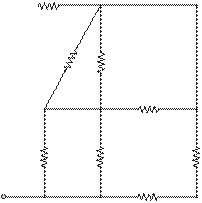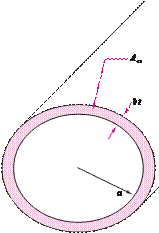### Create an Account

Home / Questions / Find the equivalent resistance between terminals a and b in the circuit of Figure P247 ...

# Find the equivalent resistance between terminals a and b in the circuit of Figure P247 3 Ù substrate

Find the equivalent resistance between terminals a

and in the circuit of Figure P2.47.

3 Ù                                                                                    substrate (see Figure P2.50). If such a cylinder hasa                                                                                                                      radius and length d, determine the thickness of the film required for a resistance if

= 1 mm                   = 33 kQ

6 Ù

12 Ù

1                 S=

 =

o               2.9M

ρ                 m

= 9 mm

4 Ù                                                 Neglect the end surfaces of the cylinder and assume that the thickness is much smaller than the radius.4 Ù               4 Ù

2 Ù

2 Ù

b

Figure P2.47

Jun 19 2020 View more View LessSubscribe To Get Solution